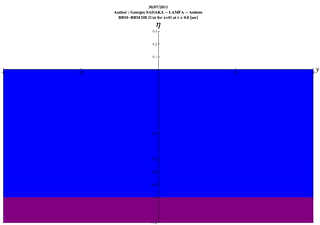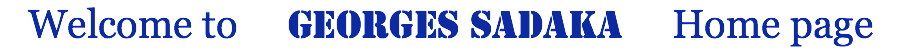Boussinesq

We consider here different family of Boussinesq systems in two space dimensions. These systems approximate the three-dimensional Euler equations and consist of three coupled nonlinear dispersive wave equations that describe propagation of long surface waves of small amplitude in ideal fluids over a horizontal bottom.

We consider first the following BBM-BBM system with a variable bottom in space -D(x,y) :we simulate the propagation of the solution in a Passive case, that looks like a Tsunami generated by an earthquake, in the Mediterranean sea with a flat bottom -D(x,y) =-1.5 km, on full domain: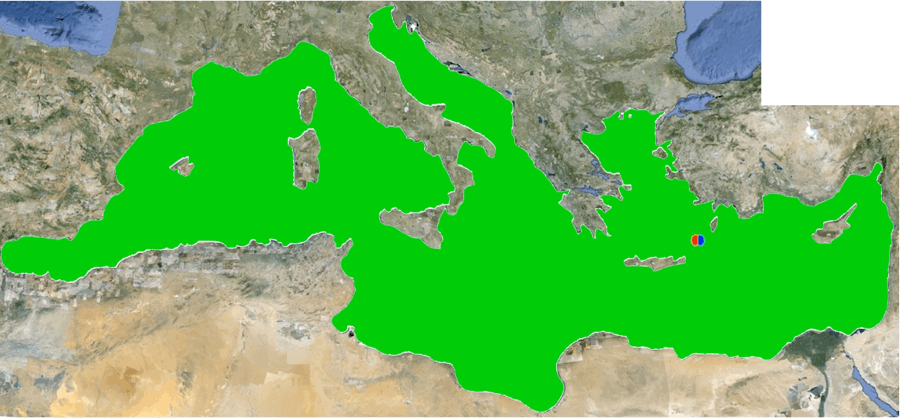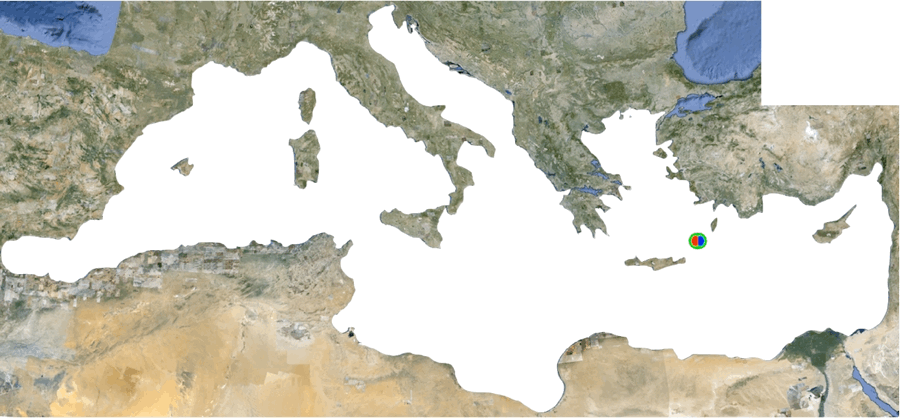Here, we consider the BBM-BBM system over a variable bottom in space D(x,y)=.25(tanh(x)-3), where reflexives boundary condition has been taken where we have wall and homogeneous Neumann boundary conditions otherwise.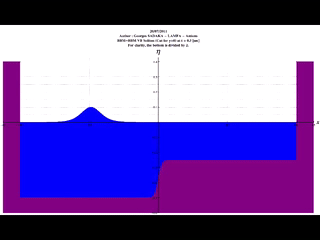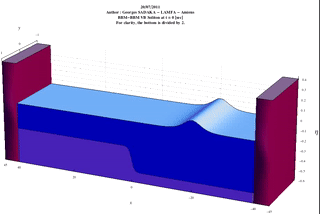Here, we simulate the propagation of a solitary wave through a port where we consider the BBM-BBM system over a flat bottom in space D(x,y)=1, with reflexives boundary condition has been taken where we have wall and homogeneous Neumann boundary conditions otherwise.Reflexion of a solitary wave over a wall for the BBM-BBM system over a flat bottom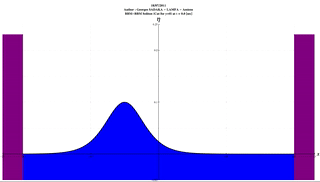Propagation of a Gaussian wave for the BBM-BBM system over a flat bottom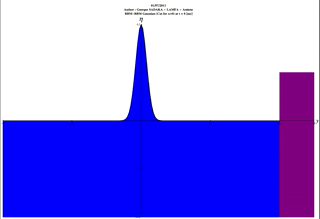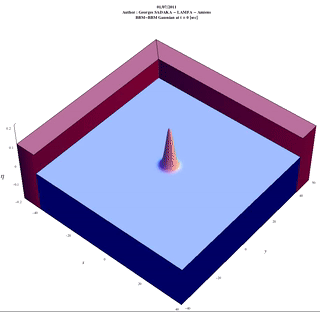Propagation of a Gaussian wave for the general Boussinesq system over a flat bottom with a=c=-1/12, b=d=1/4, D(x,y)=1.Propagation of a Gaussian wave for the Boussinesq system over a flat bottom with a=-1/3,c=0, b=d=1/3, D(x,y)=1.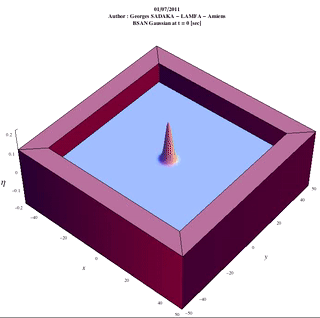Propagation of a Gaussian wave for the Bona-Smith system over a flat bottom with a=0, c=-1/3, b=d=1/3, D(x,y)=1.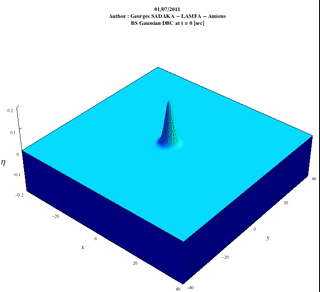Propagation of a Gaussian wave for the KdV-KdV system over a flat bottom with a=c=1/6, b=d=0, D(x,y)=1.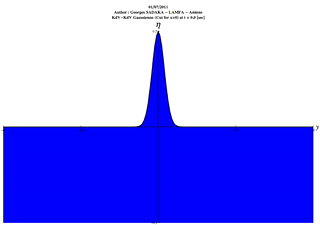Here, we consider the following BBM-BBM system over a dynamic bottom :where we used P1 finite element space, with bi-periodic boundary condition on all the boundary.